# MCQ in Clock, Variation, and Progression Problems Part 2 | ECE Board Exam

(Last Updated On: February 6, 2020)This is the Multiples Choice Questions Part 2 of the Series in Clock, Variation, and Progression Problems of the Engineering Mathematics topic. In Preparation for the ECE Board Exam make sure to expose yourself and familiarize in each and every questions compiled here taken from various sources including but not limited to past Board Examination Questions in Engineering Mathematics, Mathematics Books, Journals and other Mathematics References.

### Multiple Choice Questions Topic Outline

• MCQ in Clock Problems | MCQ in Progression / Sequence Series | MCQ in Arithmetic Progression | MCQ in Geometric Progression | MCQ in Infinite Geometric Progression | MCQ in Harmonic Progression | MCQ in Related Sequence | MCQ in Fibonacci Numbers | MCQ in Lucas Numbers | MCQ in Figurate Numbers (Triangular Numbers, Square Numbers, Gnomons, Oblong Numbers, Pentagonal Numbers, Cubic Numbers, Tetrahedral Numbers, Square Pyramidal Numbers, Supertetrahedral Numbers | MCQ in Diophantine Equations | MCQ in Variation Problems

### Continue Practice Exam Test Questions Part II of the Series

Choose the letter of the best answer in each questions.

Problem 51 (ECE November 1996)

How many terms of the sequence -9, -6, -3 … must be taken so that the sum is 66?

• A. 13
• B. 12
• C. 4
• D. 11

Problem 52 (CE November 1997)

The sum of the progression 5, 8, 11, 14 …. is 1025. How many terms are there?

• A. 22
• B. 23
• C. 24
• D. 25

Problem 53 (CE May 1998)

There are seven arithmetic means between 3 and 35. Find the sum of all terms.

• A. 169
• B. 171
• C. 167
• D. 173

Problem 54 (CE May 1999)

There are line (9) arithmetic means between 11 and 51. The sum of the progression is:

• A. 279
• B. 341
• C. 376
• D. 254

Problem 55

The sum of all even numbers from 0 to 420 is:

• A. 43410
• B. 44300
• C. 44310
• D. 44130

Problem 56 (CE May 1997)

Which of the following numbers should be changed to make all the numbers form an arithmetic progression when properly arranged?

• A. 27/14
• B. 33/28
• C. 45/28
• D. 20/14

Problem 57

The first term of an arithmetic progression (A.P.) is 6 and the 10th term is 3 times the second number. What is the common difference?

• A. 1
• B. 2
• C. 3
• D. 4

Problem 58

The sum of five arithmetic means between 34 and 42 is:

• A. 150
• B. 160
• C. 190
• D. 210

Problem 59

The positive values of a so that 4x, 5x + 4, 3×2 – 1will be in arithmetic progression is:

• A. 2
• B. 3
• C. 4
• D. 5

Problem 60

Solve for x if x + 3x + 5x + 7x + … + 49x = 625

• A. ¼
• B. ½
• C. 1
• D. 1 ¼

Problem 61

The 10th term of the series a, a – b, a – 2b, … is:

• A. a – 6b
• B. a – 9b
• C. 2a – b
• D. a + 9b

Problem 62

If the sum of the first 13 terms of two arithmetic progressions are in the ratio 7:3, find the ratio of their corresponding 7th term.

• A. 3:7
• B. 1:3
• C. 7:3
• D. 6:7

Problem 63

If 1/x, 1/y, 1/z are in arithmetic progression, then y is equal to:

• A. X-z
• B. ½(x+2z)
• C. (x+z)/2xz
• D. 2xz/(x+z)

Problem 64 (ECE November 1997)

Find the 30th term of the A.P. 4, 7, 10 …

• A. 88
• B. 91
• C. 75
• D. 90

Problem 65 (ECE November 1997)

Find the 100th term of the sequence 1.01, 1.00, 0.99….

• A. 0.05
• B. 0.04
• C. 0.03
• D. 0.02

Problem 66

The sum of all numbers between 0 and 10000 which is exactly divisible by 77 is:

• A. 546546
• B. 645568
• C. 645645
• D. 645722

Problem 67 (ME April 1998)

What is the sum of the following finite sequence of terms? 18, 25, 32, 39, .., 67.

• A. 234
• B. 181
• C. 213
• D. 340

Problem 68

Find x in the series: 1, 1/3, 0.2, x.

• A. 1/6
• B. 1/8
• C. 1/7
• D. 1/9

Problem 69 (ECE November 1995)

Find the fourth term of the progression ½, 0.2, 0.125, …

• A. 0.102
• B. 1/10
• C. 1/11
• D. 0.099

Problem 70

The 10th term of the progression 6/4, 4/3, 3/2, … is:

• A. 12
• B. 10/3
• C. 12/3
• D. 13/3

Problem 71 (ME October 1997)

The geometric mean of 4 and 64 is:

• A. 48
• B. 16
• C. 34
• D. 24

Problem 72 (ME October 1997)

The geometric mean of a and b is:

• A. √ab
• B. (a+b)/2
• C. 1/b
• D. ab/2

Problem 73 (CE May 1998)

Determine the sum of the infinite geometric series of 1, -1/5,+1/25, …?

• A. 4/5
• B. 5/7
• C. 4/6
• D. 5/6

Problem 74

There are 6 geometric means between 4 and 8748. Find the sum of all terms.

• A. 13120
• B. 15480
• C. 10250
• D. 9840

Problem 75 (ECE April 1998)

Find the sum of the infinite progression 6, -2, -2/3 …

• A. 5/2
• B. 9/2
• C. 7/2
• D. 11/2

Problem 76 (ECE April 1998)

Find the sum of the first 10 terms of the Geometric Progression 2, 4, 8, 16 …

• A. 1023
• B. 2046
• C. 1596
• D. 225

Problem 77

The 1st, 4th, 8th terms of an A.P. are themselves geometric progression (G.P.). What is the common ratio of the G.P.?

• A. 4/3
• B. 5/3
• C. 2
• D. 7/3

Problem 78

Determine x so that x, 2x + 7, 10x – 7 will form a geometric progression.

• A. -7
• B. 6
• C. 7
• D. -6

Problem 79

The fourth term of a geometric progression is 189 and the sixth term is 1701, the 8th term is:

• A. 5103
• B. 1240029
• C. 45927
• D. 15309

Problem 80

The sum of the numbers in arithmetical progression is 45. If 2 is added to the first number, 3 to the second and 7 to the third, the new numbers will be in geometrical progression. Find the common difference in A.P.

• A. -5
• B. 10
• C. 6
• D. 5

Problem 81

The geometric mean and the harmonic mean of two numbers are 12 and 36/5 respectively. What are the numbers?

• A. 36 & 4
• B. 72 & 8
• C. 36 & 8
• D. 72 & 4

Problem 82

If x, 4x + 8, 30x + 24 are in geometrical progression, find the common ratio.

• A. 2
• B. 4
• C. 6
• D. 8

Problem 83 (ECE April 1995)

A besiege fortress is held by 5700 men who have provision for 66 days. If the garrison loses 20 men each day, for how many days can the provision hold out?

• A. 60
• B. 72
• C. 76
• D. 82

Problem 84 (ECE April 1999)

If one third of the air in the tank is removed by each stroke of an air pump, what fractional part of the total air is removed in 6 strokes?

• A. 0.9122
• B. 0.0877
• C. 0.8211
• D. 0.7145

Problem 85

A rubber ball is dropped from a height of 15m. On each rebound, it rises 2/3 of the height from which it last fell. Find the distance traveled by the ball before it becomes to rest.

• A. 75 m
• B. 96 m
• C. 100 m
• D. 85 m

Problem 86 (CE May 1991)

In the recent Bosnia conflict, The NATO forces captured 6400 soldiers. The provisions on hand will last for 216 meals while feeding 3 meals a day. The provisions lasted 9 more days because of daily deaths. At an average, how many died per day?

• A. 15.2
• B. 17.8
• C. 18.3
• D. 19.4

Problem 87

To build a dam, 60 men must work 72 days. If all 60 men are employed at the start but the number is decreased by 5 men at the end of each 12-day period, how long will it take to complete the dam?

• A. 108 days
• B. 9 days
• C. 94 days
• D. 60 days

Problem 88 (CE November 1994)

In a benefit show, a number of wealthy men agreed that the first one to arrive would pay 10 centavos to enter and each later arrival would pay twice as much as the preceding man. The total amount collected from all of them was P104857.50. How may wealthy men had paid?

• A. 18
• B. 19
• C. 20
• D. 21

Problem 89

Evaluate the following determinant: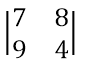• A. 64
• B. 44
• C. 54
• D. -44

Problem 90

The following equation involves two determinants: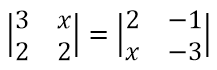The value of x is:

• A. 1
• B. 3
• C. 4
• D. 3

Problem 91 (CE November 1997)

Evaluate the following determinant: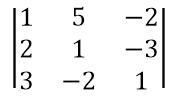• A. -24
• B. 24
• C. -46
• D. 46

Problem 92 (CE November 1996)

Compute the value of x from the following: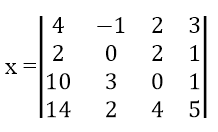• A. 27
• B. -28
• C. 26
• D. -29

Problem 93

Evaluate the following determinant: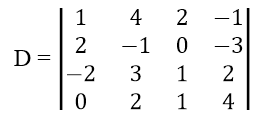• A. 5
• B. -4
• C. 4
• D. -5

Problem 94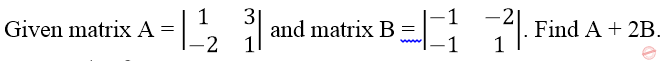Find A + 2B.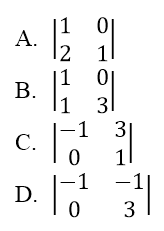Problem 95 (CE May 1996)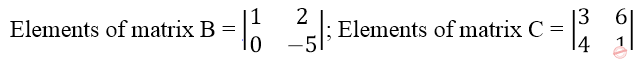Find the elements of the product of the two matrices, matrix BC.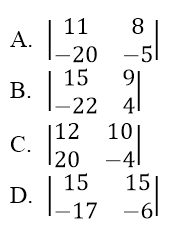Problem 96 (CE Board)

Solve for x and y from the given relationship: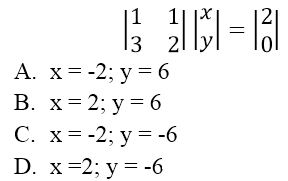• A. x = -2; y = 6
• B. x = 2; y = 6
• C. x = -2; y = -6
• D. x =2; y = -6

Problem 97 (EE October 1993)

In a class of 40 students, 27 students like Calculus and 25 like Geometry. How many students liked both Calculus and Geometry?

• A. 10
• B. 14
• C. 11
• D. 12

Problem 98

A class of 40 took examination in Algebra and Trigonometry. If 30 passed Algebra, 36 passed Trigonometry, and 2 failed in both subjects, the number of students who passed the two subjects is:

• A. 2
• B. 8
• C. 28
• D. 25

Problem 99 (ECE November 1992)

The probability for the ECE board examinees from a certain school to pass the Mathematics subject is 3/7 and that for the Communications subject is 5/7. If none of the examinees failed in both subjects and there are 4 examinees who pass both subjects, how many examinees from the school took the examination?

• A. 28
• B. 27
• C. 26
• D. 32

Problem 100 (EE March 1998)

In a commercial survey involving 1000 persons on brand preferences, 120 were found to prefer brand x only, 200 persons prefer brand y only, 150 persons prefer brand z only, 370 prefer either brand x or y not z, 450 prefer brand y or z but not x, and 370 prefer wither brand z or x but not y, and none prefer all the three brands at a time. How many persons have no brand preference with any of the three brands?

• A. 120
• B. 280
• C. 70
• D. 320

### Online Questions and Answers in Clock, Variation, and Progression Problems Series

Following is the list of multiple choice questions in this brand new series:

Clock, Variation, and Progression Problems MCQs
PART 1: MCQs from Number 1 – 50                        Answer key: PART I
PART 2: MCQs from Number 51 – 100                   Answer key: PART II
Help Me Makes a Difference!

 P inoyBIX educates thousands of reviewers/students a day in preparation for their board examinations. Also provides professionals with materials for their lectures and practice exams. Help me go forward with the same spirit. “Will you make a small \$5 gift today?” Option 1 : \$1 USD Option 2 : \$3 USD Option 3 : \$5 USD Option 4 : \$10 USD Option 5 : \$25 USD Option 6 : \$50 USD Option 7 : \$100 USD Option 8 : Other Amount© 2014 PinoyBIX Engineering. © 2019 All Rights Reserved | How to Donate? |#### GEAS Solution

Dynamics problem Economics problem Physics problem Statics problem Strength problem Thermodynamics problem

#### Questions and Answers in GEAS

Engineering Economics Engineering Laws and Ethics Engineering Management Engineering Materials Engineering Mechanics General Chemistry Physics Strength of Materials Thermodynamics
Consider Simple Act of Caring!: LIKE MY FB PAGE

Our app is now available on Google Play, Pinoybix Elex